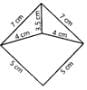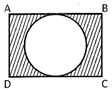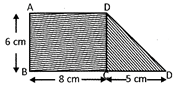# Test : Area - 2

## 10 Questions MCQ Test Mathematics for Class 5: NCERT | Test : Area - 2

Description
Attempt Test : Area - 2 | 10 questions in 10 minutes | Mock test for Class 5 preparation | Free important questions MCQ to study Mathematics for Class 5: NCERT for Class 5 Exam | Download free PDF with solutions
QUESTION: 1

### Perimeter of an isosceles triangle is 32 cm. If length of the unequal side is 13 cm, find the length of one of the equal sides of the triangle.

Solution:

Perimeter of an isoclees triangle=32.
In an isoclees triangle two sides are equal.
the another side was different
here in the question they gave the unequal side length,which is13cm=y(say).
therefore 2x+y=32
2x+13=32
2x=32-13
2x=19
X=19/2
X=9.5

QUESTION: 2

Solution:
QUESTION: 3

### Find the area of the triangle whose base is 15 cm and height is 20 cm.

Solution:
QUESTION: 4

Find the perimeter of the following figure.Solution:
QUESTION: 5

Perimeter of a square is 60 cm. Find the area of the square.

Solution:
QUESTION: 6

Look at the following figure:ABCD is a rectangle whose length and breadth are 16 cm and 14 cm respectively. Inside the rectangle ABCD, there is a circle. Find the area of the shaded region.

Solution:
QUESTION: 7

Perimeter of a circle is 1.1 cm. Find the area of the circle.

Solution:
QUESTION: 8

Base of a triangle is 2 cm more than the height. If height of the triangle is 12 cm, find the area of the triangle.

Solution:
QUESTION: 9

Perimeter of a square is equal to the perimeter of the rectangle. If length and breadth of the rectangle is 24 cm and 20 respectively. Find the area of the square.

Solution:
QUESTION: 10

Look at the following figure:ABCD is a rectangle. Find the area of the figure.

Solution:Use Code STAYHOME200 and get INR 200 additional OFF Use Coupon Code